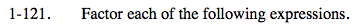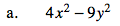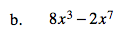### Home > PC > Chapter 1 > Lesson 1.3.2 > Problem1-121

1-121.
1. Factor each of the following expressions. Homework Help ✎

1. 4x2 − 9y2

2. 8x3 − 2x7This is the difference of two perfect squares.

(2x - 3y)(2x + 3y)Factor out the common factors first.

$2x^3(4-x^4)$

Now you have a difference of two perfect squares. Factor again.